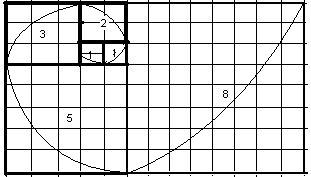# Golden Ratio Calculator

Calculator finds the values that satisfy the golden ratio where (A+B):A = A:B.

Please Enter any ratio term to calculate the missing golden ratio values.

 A+B : A : A : B :

## Examples

Example 1: We enter the value for (A + B) = 5 and A = 3 (in both place)

The calculator finds the missing value for B and display 2

Example 2: We enter the value for (A + B) = 51 and A = 3 (in both place)

The calculator finds the missing value for B and display 2 and also correct the value enter (A + B) = 51 as 5

### Importance of Golden Ratio

The golden ratio is a mathematical ratio which is commonly found in nature. The golden ratio is used in our day-to-day lives, art, and architecture.

The objects designed to reflect the golden ratio in their structure such design is more pleasing , gives an aesthetic feel to the eyes which can be noticed in the spiral arrangement of flowers and leaves.

In mathematics, two given quantities are in the golden ratio if their ratio is the same as the ratio of their sum to the larger of the two quantities.

The Golden Ratio or golden mean, or golden section describes the perfectly symmetrical relationship between two proportions. It is also known as divine proportion.

If the larger quantity divided by smaller quantity is equal to the sum of two quantities divided by the larger quantity than given quantities are called in golden ratio.

In the Fibonacci Sequence (0, 1, 1, 2, 3, 5, 8, 13, ...) each term is the sum of the previous two, and the ratio becomes increasingly closer to the Golden Ratio.

The formula for Golden Ratio

= ½(1 + √5) or 1.6180339887499.

A/B = (A+B)/A = 1.6180339887499.